# Fractal-like structures arising from the action of a group on $\mathbb{Z}^2$

Let $G := \langle a, b, c \rangle < {\rm Sym}(\mathbb{Z}^2)$ be the group generated by the permutation $$a: \ (m,n) \ \mapsto \ (m-n,m)$$ of order $6$ and the involutions $$b: \ (m,n) \ \mapsto \ \begin{cases} (m,2n+1) & \text{if} \ n \equiv 0(\text{mod} \ 2), \\ (m,(n-1)/2) & \text{if} \ n \equiv 1(\text{mod} \ 4), \\ (m,n) & \text{if} \ n \equiv 3(\text{mod} \ 4) \\ \end{cases}$$ and $$c: \ (m,n) \ \mapsto \ \begin{cases} (m,2n+3) & \text{if} \ n \equiv 0(\text{mod} \ 2), \\ (m,(n-3)/2) & \text{if} \ n \equiv 3(\text{mod} \ 4), \\ (m,n) & \text{if} \ n \equiv 1(\text{mod} \ 4). \\ \end{cases}$$ Drawing spheres of radius $r$ about $(0,0)$ for "large enough" $r$ reveals fractal-like structures.

Added on July 28, 2014: A video showing more pictures is now available on YouTube here. The video starts with a sequence showing entire spheres of small radii, i.e. from $r = 8$ to $r = 24$, and continues with pictures showing smaller parts of spheres of larger radii up to $r = 45$. Monochrome pictures show only one sphere, respectively, a part thereof; colored pictures show multiple spheres in different colors.

Added on March 16, 2014: Pictures of the spheres of radius $30$ and $36$ can be downloaded here:

Sample snippets of the large -- about $200$ megapixels at $r = 38$ to about $3$ gigapixels at $r = 45$ -- pictures are (black pixel = belongs to sphere, white pixel = doesn't belong to sphere):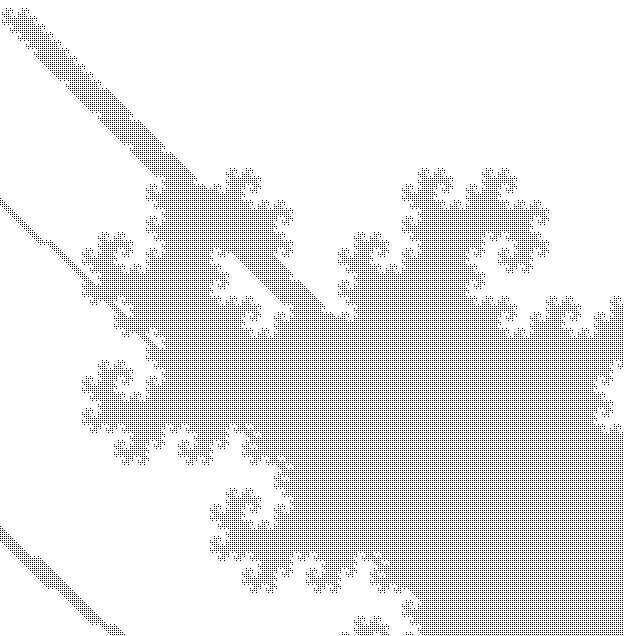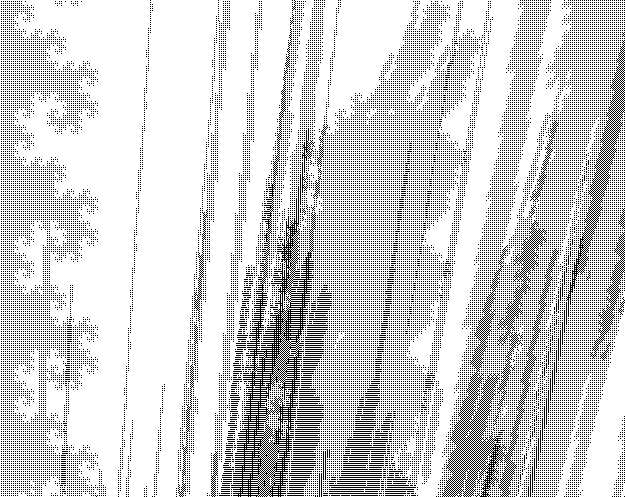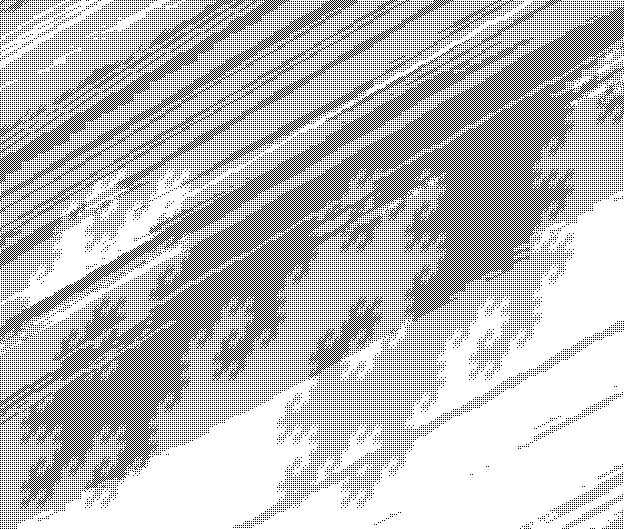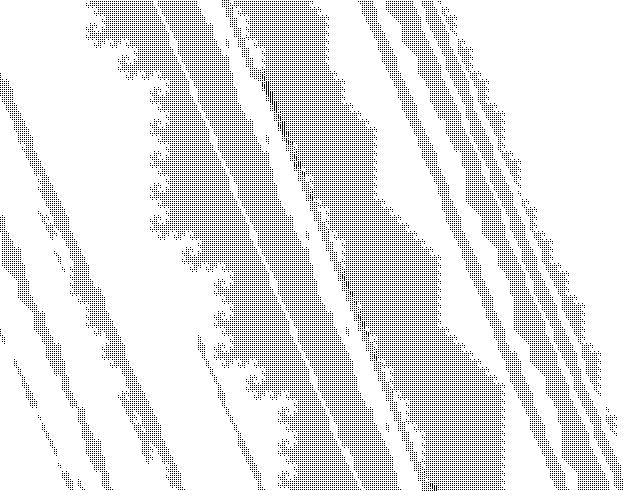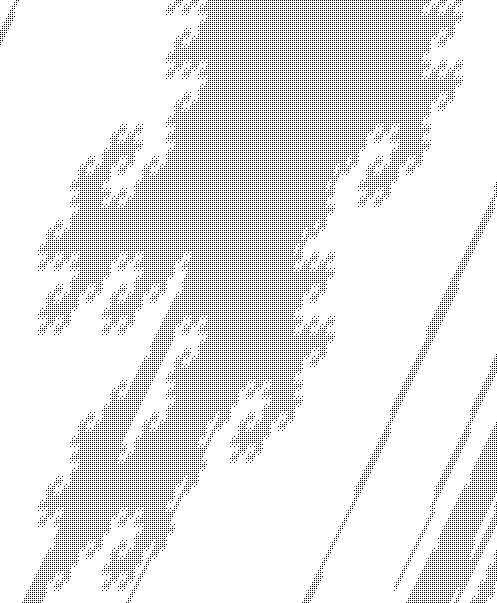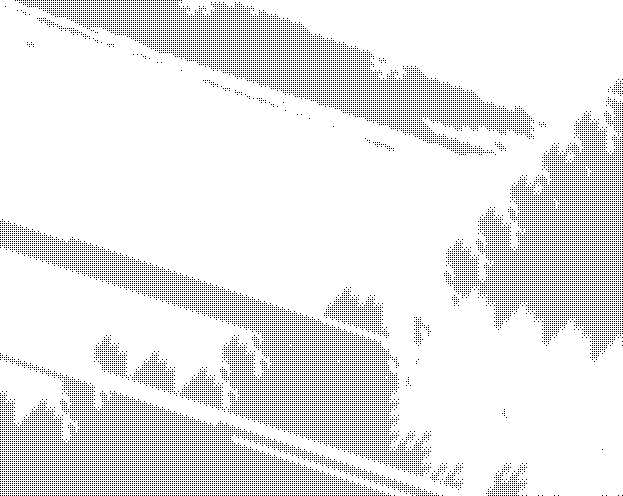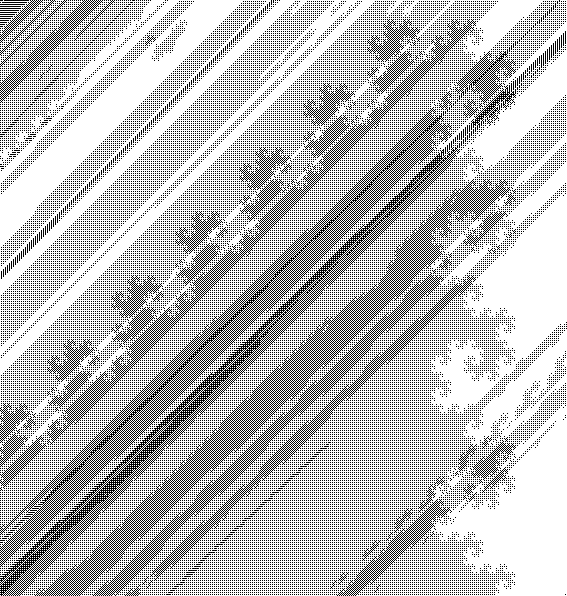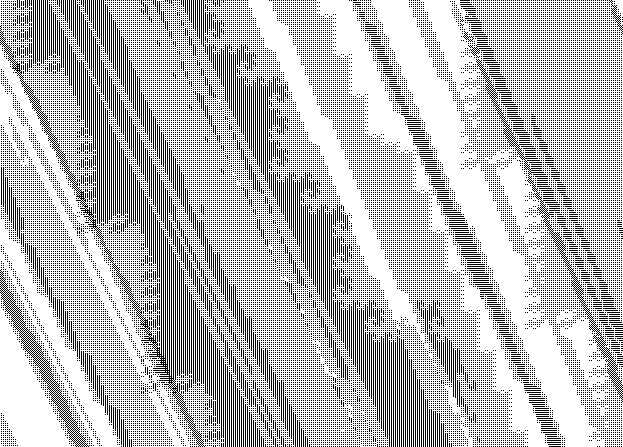The images above show parts of the spheres of radius $38$, $40$ and $45$.

Question: How can the observed patterns be explained?

Remark 1: The cardinalities of the spheres of radii $r = 0, \dots, 45$ about $(0,0)$ are

1, 2, 4, 8, 14, 26, 39, 68, 114, 188, 289, 404, 560, 827, 1341, 2052, 3158, 4540,
6091, 8630, 12241, 17739, 27727, 41846, 61234, 86647, 117806, 163795, 233939, 340659,
523862, 768739, 1110855, 1569204, 2148377, 2994661, 4287462, 6195498, 9389566,
13568954, 19542862, 27619364, 38048372, 53304607, 76433012, 109839303.


The entire sphere of radius $20$ looks as follows (the overall shape of the larger spheres is roughly similar):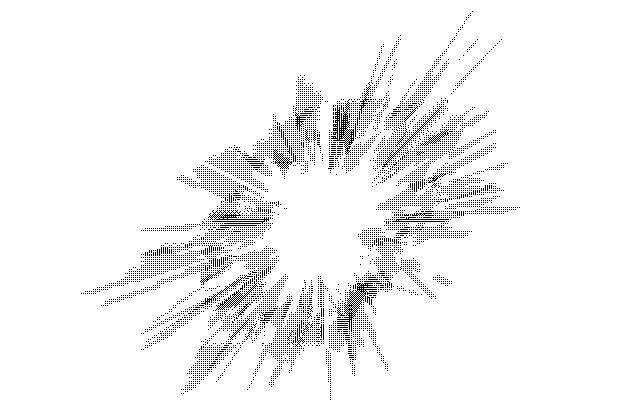Added: At a scale of about $1:100$, the entire sphere of radius $45$ looks as follows: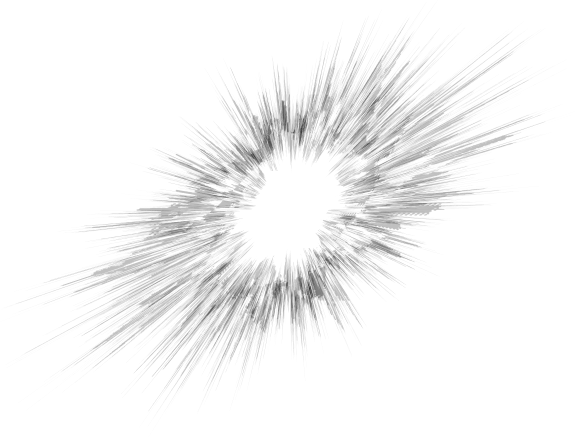Remark 2: In the notation of this and this question, $b$ and $c$ induce on the second coordinate the class transpositions $\tau_{0(2),1(4)}$ and $\tau_{0(2),3(4)}$, respectively. Further, in the notation of (1) and (2) we have $G < {\rm RCWA}(\mathbb{Z}^2)$.

• Hm, what do you mean by the sphere here? You are looking at the forward orbit of (0,0) under all maps, are you not? Mar 11, 2014 at 20:00
• This is a wonderful question! Mar 12, 2014 at 2:48
• Why don't you put the tags ds.dynamical-systems and fractals ? Experts of these subjects could help you (there are others fractal-like phenomenons on discrete strutures, for example the Rauzy fractals). Mar 12, 2014 at 12:51
• Besides the fractal structure, the spheres look like to an explosion, I had never seen such pattern in pure mathematics. I don't know if there is something to say mathematically about that, perhaps such group actions appear in a discrete modelling of physical explosions. Mar 14, 2014 at 18:45
• What beautiful pictures! An animation showing successively all the spheres from radius 0 to radius 45 would be interesting too: one animation with a fixed scale, and one with a renormalized scale. Do you observe that the shape of the spheres (at renormalized scale) "converge" to a (unique) fractal shape (I hope my question is understandable) ? Mar 17, 2014 at 11:31

This phenomenon can be understood as follows:

Let $\Gamma < Sym(\mathbb{Z}^{2})$ be a transitive hyperbolic group (and non virtually cyclic).

Through this faithful transitive action of $\Gamma$ on $\mathbb{Z}^{2}$ (and orbit of (0,0)), the Cayley graph of $\Gamma$ (Gromov hyperbolic geometry) is projected (in a manner not too degenerate) on $\mathbb{Z}^2$ (euclidean geometry).
Then the emergence of explosive and fractal-like structures on the spheres is not surprising.

So any (non virtually cyclic) hyperbolic group would generate beautiful fractal-like pictures.

Example: $\Gamma = \langle a,b \rangle < Sym(\mathbb{Z}^{2})$ such that

• $a: (m,n) \to (m+1,m+n)$
• $b: (m,n) \to (m+n,n+1)$

To be confirmed: $\Gamma$ acts transitively on $\mathbb{Z}^{2}$.
Remark: $\Gamma$ is neither free nor hyperbolic nor torsion-free (see the comments below).

The cardinalities of the spheres of radii $r=0,\dots,14$ about $(0,0)$ are :
$1,4,11,20,47,100,238,512,1124,2540,5569,12101,26208,56720,122600$

The entire sphere of radius $14$:The entire ball of radius $14$ with a rainbow gradient according to the spheres:Remark: I don't know if your group $G$ is hyperbolic, but we could have the same understanding for all the finitely generated groups of exponential growth.

• Nice picture! -- In fact, there are many diffferent possibilities how these pictures may look like for a particular group -- for some groups one just gets more-or-less random-looking ring-shaped pixel clouds, for others one gets various geometric patterns with varying complexity, and for some groups one observes fractal-like patterns like for the group my question is about. Some much simpler examples are shown here: gap-system.org/DevelopersPages/StefanKohl/rcwa/pictures.html Mar 18, 2014 at 0:02
• @StefanKohl: These pictures are not defined as a non-escaping set of a complex function (because the group acts transitively...), nevertheless I ask myself if there is a way to define a transformation such that its non-escaping set is exactly the renormalized limit of the spheres. What do you think? Also, by taking $\Gamma<Sym(\mathbb{Z}^3)$ (non virtually cyclic) transitive hyperbolic, one should obtain nice 3D fractals! There is an expert in mathematical imagery: Jos Leys, he often works with Etienne Ghys. Mar 18, 2014 at 16:45
• This one vaguely resembles the s. c. "Farey sunbursts" obtained by joining consecutive $(m,n)$s corresponding to the $m/n$s in the Farey sequence... Mar 21, 2014 at 11:00
• In fact this particular group is not free: for example, $$ba^{-1}bab^{-1}a(m,n)=a^{-1}bab^{-1}ab(m,n)=(m+2,n).$$ Still most likely it is hyperbolic... Mar 21, 2014 at 17:26
• @მამუკაჯიბლაძე: By your first comment, the element $g_1:(m,n) \to (m+2,n)$ is in $\Gamma$. By symmetry, $g_2:(m,n) \to (m,n+2)$ is also in $\Gamma$. But $\langle g_1 , g_2 \rangle \simeq \mathbb{Z}^2$. Then $\mathbb{Z}^2$ is a subgroup of $\Gamma$, and so $\Gamma$ is not hyperbolic. But $\mathbb{Z}^2 \triangleleft \Gamma$, so perhaps $\Gamma / \mathbb{Z}^2$ is hyperbolic. Mar 21, 2014 at 23:26

This is just a partial answer, but it looks like your figures are not "pure" fractals, but a union of different fractals (with different fractal dimensions). If I am not mistaken, these are called multi-fractals.

It looks like part of your figure is the Dragon curve fractal.

This particular fractal is space-filling, so it would not surprise me if your set is actually everything, (unless I have misinterpreted something).

Also, your dynamics looks a lot like some two-dimensional version of the iterations of something similar to the Collatz conjecture, which give rise to a fractal behaviour. Look at the corresponding wikipedia page, there is an analytic continuation of the functions that are iterated, and I think something similar can be done in your case.

Ok, so you can make a continuous version of your map $b$: Let $s(n)=\frac12 \sin\frac{\pi n}{2} (1 + \sin \frac{\pi n}{2})$. Note that $s(n)$ is periodic, with period 4: $1,0,0,0,1,0,\dots$.

Now, we can define $b(m,n)$ as

$$b(m,n)= \left(m, (2 n + 1) (s[n + 1] + s[n + 3]) + s[n + 2]n + s[n] (n - 1)/2 \right)$$ and this is now a continuous extension of your map, seen as a map on $\mathbb{R}^2$. You can do a similar thing with the other maps.

Now it might be easier to draw this, for example, this is exactly the kind of maps that the most common fractal drawing software works with. What you have is a Hutchinson operator, so check wikipedia again.

• As the group $G$ acts transitively on $\mathbb{Z}^2$, the orbit of $(0,0)$ under the action of $G$ is actually $\mathbb{Z}^2$, i.e. "everything" -- thus pictures of this would be pretty boring (everything black). But what my question considers are spheres (not balls!) of certain radii $r$ about $(0,0)$ -- these consist of the points which can be reached from $(0,0)$ by applying $r$ generators or inverses of generators, but no fewer. In particular, any sphere is finite. Mar 11, 2014 at 20:30
• Ah, I see! Well, still, the Dragon fractal is where I'd start. Mar 11, 2014 at 20:46
• Interesting idea! Btw your expression can be rewritten as $$b(m,n)=(m,2n+1-\frac{n+1}4(5+\sin\frac{\pi n}2)\sin^2\frac{\pi n}2).$$ Not that it is much simpler but still... Mar 23, 2014 at 9:33
• As for $c$, we could replace it with $bcb$ which is $$bcb(m,n)=(m,n+2\,\sin\frac{\pi n}2)$$ Mar 23, 2014 at 9:54
• Still slightly simpler expression for $b$: $$b(m,n)=(m,\frac{n+1}4(8-\sin\frac{\pi n}2-5\,\sin^2\frac{\pi n}2)-1)$$ Mar 24, 2014 at 7:26